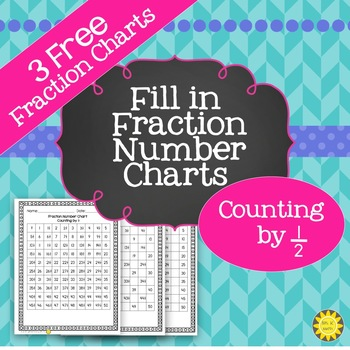# Fil in Fraction Number Charts for ½ SamplerSubject
Resource Type
File Type
PDF (3 MB|6 pages)
Standards
FREE
• Product Description
• Standards

This variety of number charts has been created for the purpose of helping students improve counting by unit fractions.

There are 3 free number charts included, which count by 1/2. There is filled in number chart and 2 number charts with missing spaces to fill in

There are 2 levels of fill in number charts are successively more difficult. These fill-in charts could be used for scaffolded practice or for differentiating levels.

Filling in the number charts will probably not take an entire hour class period. Guiding the students into a discussion about vertical, horizontal, and diagonal number patterns before and after filling out a number chart will be most valuable to the students in developing recognition of number patterns.

There charts would also be great for morning work after practice with counting by unit fractions.

These charts are a sampler of a larger pack of 60 number charts, which are available here .. The larger pack contains fractions of ½, ⅓, ¼, 1/5, 1/6, ⅛, 1/10, 1/12, and 1/100.

Understand a fraction 𝘢/𝘣 as a multiple of 1/𝘣. For example, use a visual fraction model to represent 5/4 as the product 5 × (1/4), recording the conclusion by the equation 5/4 = 5 × (1/4).
Apply and extend previous understandings of multiplication to multiply a fraction by a whole number.
Compare two fractions with different numerators and different denominators, e.g., by creating common denominators or numerators, or by comparing to a benchmark fraction such as 1/2. Recognize that comparisons are valid only when the two fractions refer to the same whole. Record the results of comparisons with symbols >, =, or <, and justify the conclusions, e.g., by using a visual fraction model.
Understand two fractions as equivalent (equal) if they are the same size, or the same point on a number line.
Explain equivalence of fractions in special cases, and compare fractions by reasoning about their size.
Total Pages
6 pages